# Top Techniques to Handle Missing Values Every Data Scientist Should Know

Explore various techniques to efficiently handle missing values and their implementations in Python.
Jan 2023  · 15 min read

Dealing with missing data is a common and inherent issue in data collection, especially when working with large datasets. There are various reasons for missing data, such as incomplete information provided by participants, non-response from those who decline to share information, poorly designed surveys, or removal of data for confidentiality reasons.

When not appropriately handled, missing data can bias the conclusions of all the statistical analyses on the data, leading the business to make wrong decisions.

This article will focus on some techniques to efficiently handle missing values and their implementations in Python. We will illustrate the benefits and drawbacks of each technique to help you choose the right one for a given situation.

## Identifying Missing Data

Missing data occurs in different formats. This section explains the different types of missing data and how to identify them.

### Types of missing data

There are three main types of missing data: (1) Missing Completely at Random (MCAR), (2) Missing at Random (MAR), and (3) Missing Not at Random (MNAR).

It is important to have a better understanding of each one for choosing the appropriate methods to handle them.

#### 1) MCAR - Missing completely at random

This happens if all the variables and observations have the same probability of being missing. Imagine providing a child with Lego of different colors to build a house. Each Lego represents a piece of information, like shape and color. The child might lose some Legos during the game. These lost legos represent missing information, just like when they can’t remember the shape or the color of the Lego they had. That information was lost randomly, but they do not change the information the child has on the other Legos.

#### 2) MAR - Missing at random

For MAR, the probability of the value being missing is related to the value of the variable or other variables in the dataset. This means that not all the observations and variables have the same chance of being missing. An example of MAR is a survey in the Data community where data scientists who do not frequently upgrade their skills are more likely not to be aware of new state-of-the-art algorithms or technologies, hence skipping certain questions. The missing data, in this case, is related to how frequently the data scientist upskills.

#### 3) MNAR- Missing not at random

MNAR is considered to be the most difficult scenario among the three types of missing data. It is applied when neither MAR nor MCAR apply. In this situation, the probability of being missing is completely different for different values of the same variable, and these reasons can be unknown to us. An example of MNAR is a survey about married couples. Couples with a bad relationship might not want to answer certain questions as they might feel embarrassed to do so.

### Methods for identifying missing data

There are multiple methods that can be used to identify missing data in pandas. Below are the most recurrent ones.

 Functions Descriptions .isnull() This function returns a pandas dataframe, where each value is a boolean value True if the value is missing, False otherwise. .notnull() Similarly to the previous function, the values for this one are False if either NaN or None value is detected. .info() This function generates three main columns, including the “Non-Null Count” which shows the number of non-missing values for each column. .isna() This one is similar to isnull and notnull. However it shows True only when the missing value is NaN type.

Table of methods for identifying missing data

## Handling Missing Data

Multiple approaches exist for handling missing data. This section covers some of them along with their benefits and drawbacks.

To better illustrate the use case, we will be using the Loan Data available on DataCamp Workspace along with the source code covered in the tutorial

Since the dataset does not have any missing values,  we will use a subset of the data (100 rows) and then manually introduce missing values.

``import pandas as pd``
``````sample_customer_data = pd.read_csv("data/customer_churn.csv",  nrows=100)
sample_customer_data.info()``````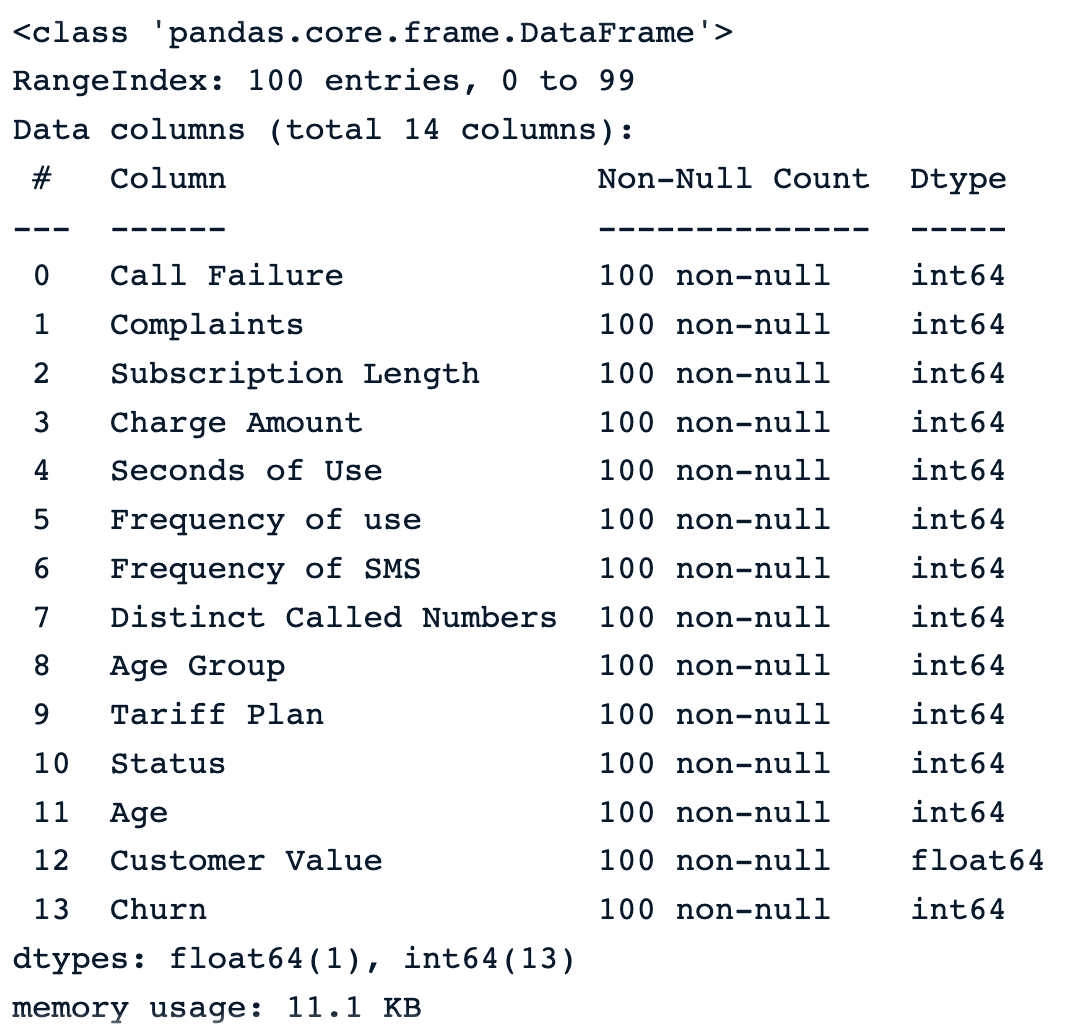Sample of 100 random samples before introducing missing values

Let’s introduce 50% of missing values in each column of the dataframe using.

``````import numpy as np
def introduce_nan(x,percentage):
n = int(len(x)*(percentage - x.isna().mean()))
idxs = np.random.choice(len(x), max(n,0), replace=False, p=x.notna()/x.notna().sum())
x.iloc[idxs] = np.nan``````

Applying the function to the data generates this result.

``````sample_customer_data.apply(introduce_nan, percentage=.5)
sample_customer_data.info()``````Sample of 100 random samples after introducing missing values

Below are the first five rows of the dataset.

``sample_customer_data.head()``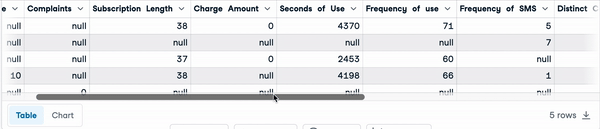First five rows with null values

### Data Dropping

Using the `dropna()` function is the easiest way to remove observations or features with missing values from the dataframe. Below are some techniques.

1) Drop observations with missing values

These three scenarios can happen when trying to remove observations from a data set:

• `dropna()`: drops all the rows with missing values.
``````drop_na_strategy = sample_customer_data.dropna()
drop_na_strategy.info()``````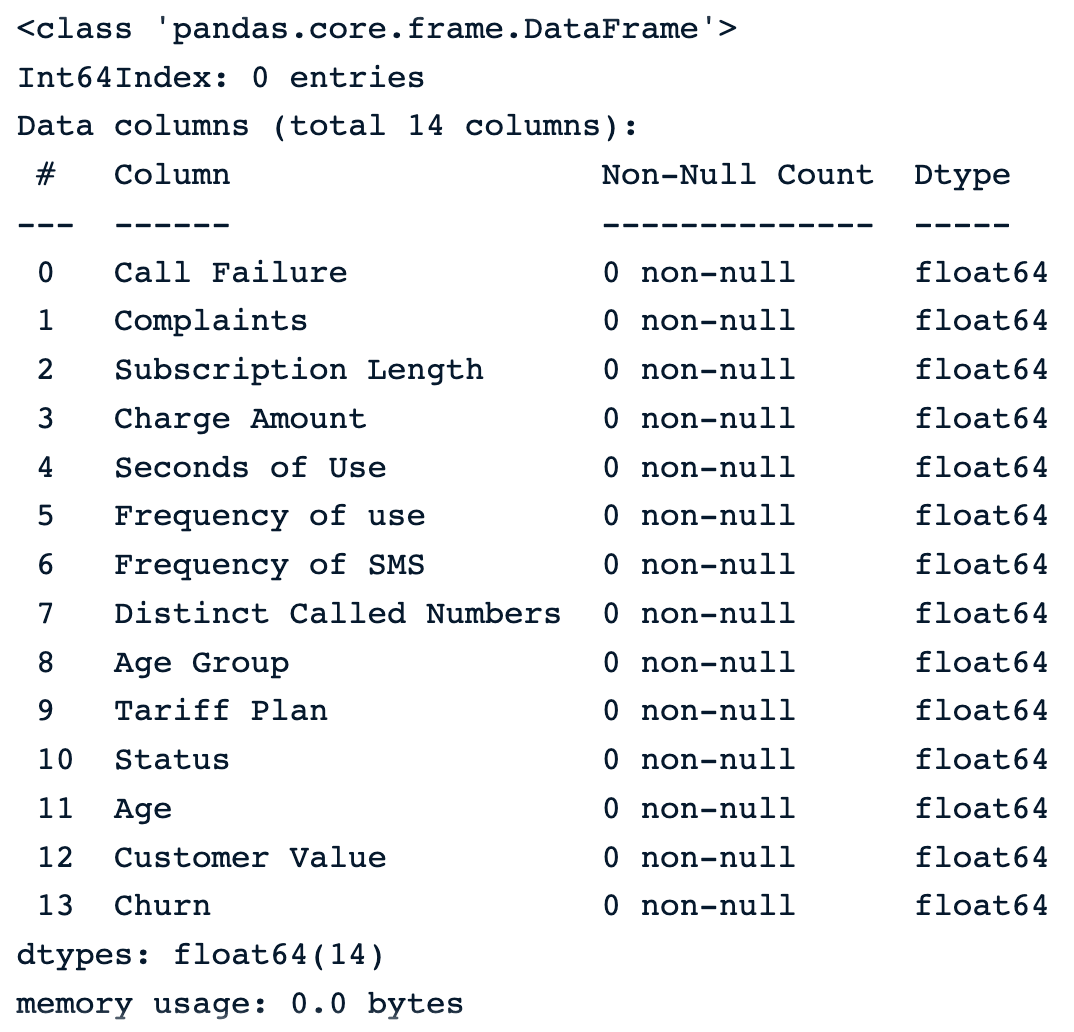Drop observations using the default `dropna()` function

We can see that all the observations are dropped from the dataset, which can be especially dangerous for the rest of the analysis.

• `dropna(how = ‘all’)`: the rows where all the column values are missing.
``````drop_na_all_strategy = sample_customer_data.dropna(how="all")
drop_na_all_strategy.info()``````

From the output below, we notice there is no observation with all the columns missing.Drop observations using the “all” strategy

• `dropna(thresh = minimum_value)`: drop rows based on a threshold. This strategy sets a minimum number of missing values required to preserve the rows.
``````drop_na_thres_strategy = sample_customer_data.dropna(thresh=0.6)
drop_na_thres_strategy.info()``````

Setting the threshold to 60%, the result is the same compared to the previous one.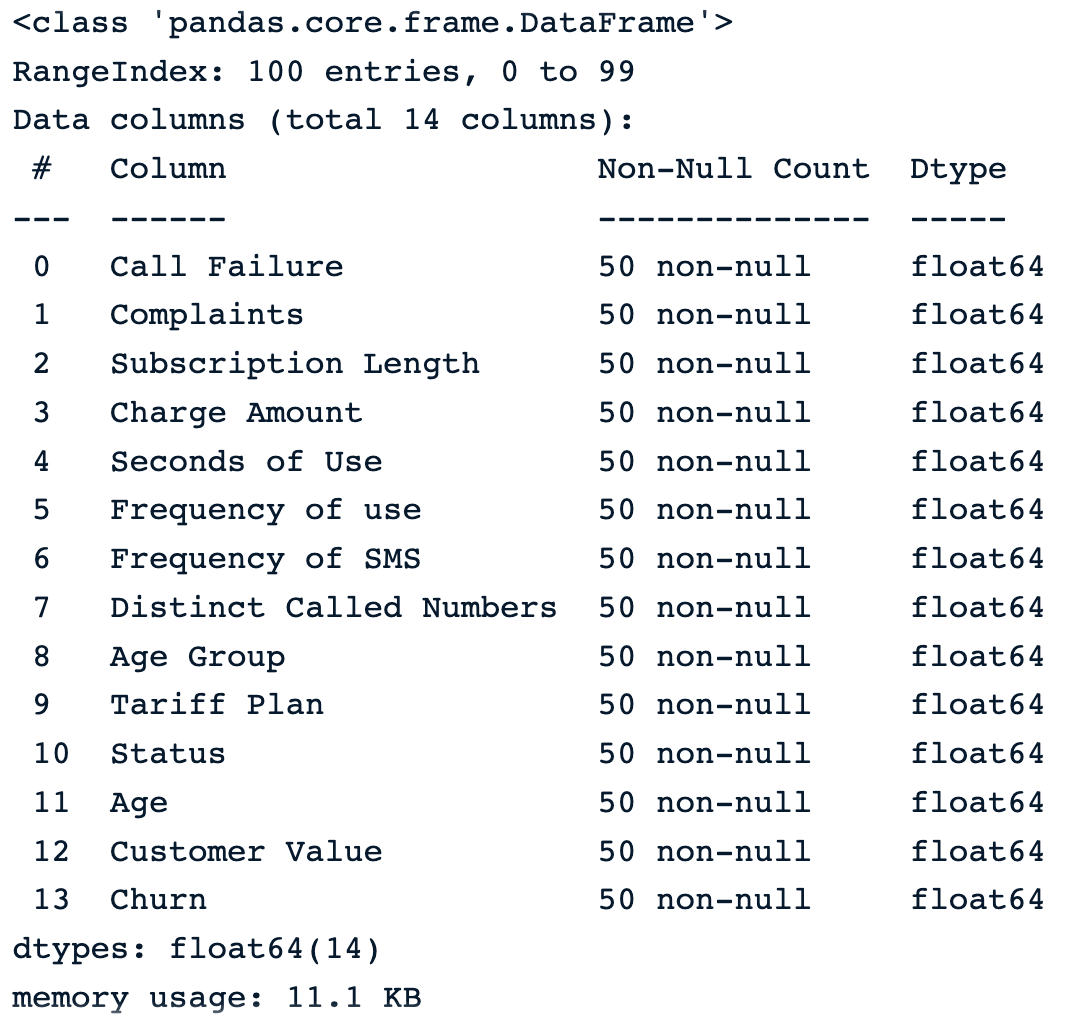Drop observations using threshold

2) Drop columns with missing values

The parameter `axis = 1` can be used to explicitly specify we are interested in columns rather than rows.

• `dropna(axis = 1)`: drops all the columns with missing values.
``````drop_na_cols_strategy = sample_customer_data.dropna(axis=1)
drop_na_cols_strategy.info()``````

There are no more columns in the data. This is because all the columns have at least one missing value.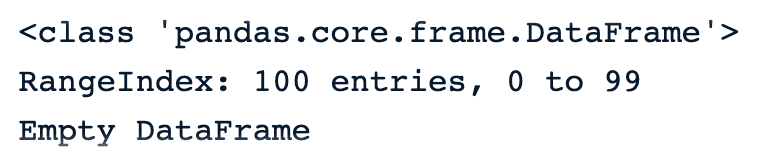Empty dataframe after `dropna()` on columns

Like many other approaches, `dropna()` also has some pros and cons.

#### Pros

• Straightforward and simple to use.
• Beneficial when missing values have no importance.

#### Cons

• Using this approach can lead to information loss, which can introduce bias to the final dataset.
• This is not appropriate when the data is not missing completely at random.
• Data set with a large proportion of missing value can be significantly decreased, which can impact the result of all statistical analysis on that data set.

### Mean/Median Imputation

These replacement strategies  are self-explanatory. Mean and median imputations are respectively used to replace missing values of a given column with the mean and median of the non-missing values in that column.

Normal distribution is the ideal scenario. Unfortunately, it is not always the case. This is where the median imputation can be helpful because it is not sensitive to outliers.

In Python, the `fillna()` function from pandas can be used to make these replacements.

• Illustration of mean imputation.
``````mean_value = sample_customer_data.mean()
mean_imputation = sample_customer_data.fillna(mean_value)``````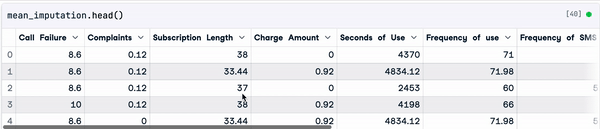Result of the mean imputation

• Illustration of median imputation
``````median_value = sample_customer_data.median()
median_imputation = sample_customer_data.fillna(median_value)Result of the median imputation

#### Pros

• Simplicity and ease of implementation are some of the benefits of the mean and median imputation.
• The imputation is performed using the existing information from the non-missing data; hence no additional data is required.
• Mean and median imputation can provide a good estimate of the missing values, respectively for normally distributed data, and skewed data.

#### Cons

• We cannot apply these two strategies to categorical columns. They can only work for numerical ones.
• Mean imputation is sensitive to outliers and may not be a good representation of the central tendency of the data. Similarly to the mean, the median also may not better represent the central tendency.
• Median imputation makes the assumption that the data is missing completely at random (MCAR), which is not always true.

### Random Sample Imputation

The idea behind the random sample imputation is different from the previous ones and involves additional steps.

• First, it starts by creating two subsets from the original data.
• The first subset contains all the observations without missing data, and the second one contains those with missing data.
• Then, it randomly selects from each subset a random observation.
• Furthermore, the missing data from the previously selected observation is replaced with the existing ones from the observation having all the data available.
• Finally, the process continues until there is no more missing information.
``````def random_sample_imputation(df):

cols_with_missing_values = df.columns[df.isna().any()].tolist()

for var in cols_with_missing_values:

# extract a random sample
random_sample_df = df[var].dropna().sample(df[var].isnull().sum(),
random_state=0)
# re-index the randomly extracted sample
random_sample_df.index = df[
df[var].isnull()].index

# replace the NA
df.loc[df[var].isnull(), var] = random_sample_df

return df``````
``````df = sample_customer_data.copy()
random_sample_imp_df = random_sample_imputation(sample_customer_data)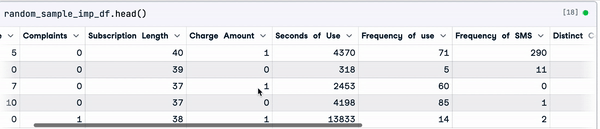Random sample imputation

#### Pros

• This is an easy and straightforward technique.
• It tackles both numerical and categorical data types.
• There is less distortion in data variance, and it also preserves the original distribution of the data, which is not the case for mean, median, and more.

#### Cons

• The randomness does not necessarily work for every situation, and this can infuse noise in the data, hence leading to incorrect statistical conclusions.
• Similarly to the mean and median, this approach also assumes that the data is missing completely at random (MCAR).

### Multiple Imputation

This is a multivariate imputation technique, meaning that the missing information is filled by taking into consideration the information from the other columns.

For instance, if the income value is missing for an individual, it is uncertain whether or not they have a mortgage. So, to determine the correct value, it is necessary to evaluate other characteristics such as credit score, occupation, and whether or not the individual owns a house.

Multiple Imputation by Chained Equations (MICE for short) is one of the most popular imputation methods in multivariate imputation. To better understand the MICE approach, let’s consider the set of variables X1, X2, … Xn, where some or all have missing values.

The algorithm works as follows:

• For each variable, replace the missing value with a simple imputation strategy such as mean imputation, also considered as “placeholders.”
• The “placeholders” for the first variable, X1, are regressed by using a regression model where X1 is the dependent variable, and the rest of the variables are the independent variables. Then X2 is used as dependent variables and the rest as independent variables. The process continues as such until all the variables are considered at least once as the dependent variable.
• Those original “placeholders” are then replaced with the predictions from the regression model.
• The replacement process is repeated for a number of cycles which is generally ten, according to Raghunathan et al. 2002, and the imputation is updated at each cycle.
• At the end of the cycle, the missing values are ideally replaced with the prediction values that best reflect the relationships identified in the data.

The implementation is performed using the miceforest library.

First, we need to install the library using the `pip`.

``pip install miceforest``

Then we import the `ImputationKernel` module and create the kernel for imputation.

``````from miceforest import ImputationKernel

mice_kernel = ImputationKernel(
data = sample_customer_data,
save_all_iterations = True,
random_state = 2023
)``````

Furthermore, we run the kernel on the data for two iterations, and finally, create the imputed data.

``````mice_kernel.mice(2)
mice_imputation = mice_kernel.complete_data()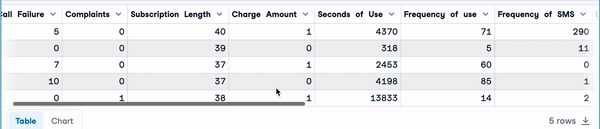Multiple imputation

#### Pros

• Multiple imputation is powerful at dealing with missing data in multiple variables and multiple data types.
• The approach can produce much better results than mean and median imputations.
• Many other algorithms, such as K-Nearest Neighbors, Random forest, and neural networks, can be used as the backbone of the multiple imputation prediction for making predictions.

#### Cons

• Multiple imputation assumes that the data is missing at random (MAR).
• Despite all the benefits, this approach can be computationally expensive compared to other techniques, especially when working with large datasets.
• This approach requires more effort than the previous ones.

From all the imputations, it is possible to identify which one is closer to the distribution of the original data.

The mean (in yellow) and median (in red) are far away from the original distribution of the “Charge amount” column data, hence are not considered to be great for imputing the data.

``````mean_imputation["Charge Amount Mean Imp"] = mean_imputation["Charge Amount"]
median_imputation["Charge Amount Median Imp"] = median_imputation["Charge Amount"]
random_sample_imp_df["Charge Amount Random Imp"] = random_sample_imp_df["Charge Amount"]``````

With the new columns created for each type of imputation, we can now plot the distribution.

``````import matplotlib.pyplot as plt
plt.figure(figsize=(12,8))

sample_customer_data["Charge Amount"].plot(kind='kde',color='blue')
mean_imputation["Charge Amount Mean Imp"].plot(kind='kde',color='yellow')
median_imputation["Charge Amount Median Imp"].plot(kind='kde',color='red')``````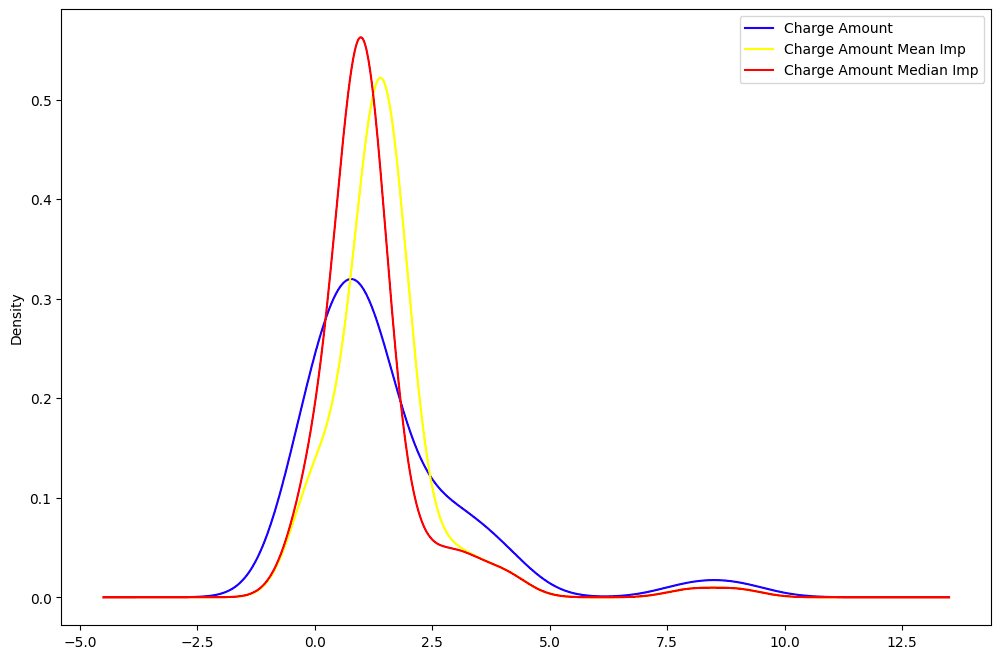Charge Amount” distribution: original data vs. mean vs. median.

Plotting the multiple imputation and the random imputation below, these distributions are perfectly overlapped with the original data. This means that those imputations are better than the mean and median imputations.

``````random_sample_imp_df["Charge Amount Random Imp"] = random_sample_imp_df["Charge Amount"]
mice_imputation["Charge Amount MICE Imp"] = mice_imputation["Charge Amount"]

mice_imputation["Charge Amount MICE Imp"].plot(kind='kde',color='black')
random_sample_imp_df["Charge Amount Random Imp"].plot(kind='kde',color='purple')

plt.legend()``````Charge Amount” distribution: original data vs. mean vs. median vs. MICE vs.random

The Handling Missing Data with Imputations in R course is a great resource to learn more about strategies to handle missing values. It covers how to apply visualization and statistical tests to recognize missing data patterns and how to impute them with both statistical and machine learning technics.

On the same note, the dealing with missing data in python course explains how to identify, analyze, remove, and impute missing data in Python.

## Best Practices

### Choosing the right imputation method based on the type of missing data

There are multiple imputation strategies, and they should not be used blindly. Adopting the right approach can save from introducing bias in the data and making wrong decisions.

The following table illustrates which imputation method to use based on the type of missing data. The list of methods is not exhaustive, but these are the most commonly used.

 Type of missing data Imputation method Missing Completely At Random Mean, Median, Mode, or any other imputation method Missing At Random Multiple imputation, Regression imputation Missing Not At Random Pattern Substitution, Maximum Likelihood estimation

### Assessing the impact of imputation on the overall analysis

It is important to keep in mind that the original data can not be recovered no matter the imputation technique. However, it is possible to use techniques that can generate imputed data sets that are as close as possible to reality.

Below are a few key steps to consider during the assessment.

• Run multiple imputation techniques to identify the most robust one. This can help identify any bias and variations from one technique to another.
• Compare the final imputed data to the original non-imputed data to assess the reliability of the imputation method.
• Include the imputation process in the overall analysis pipeline from data cleaning to building any machine learning model.

### Communicating missing data and imputation methods to stakeholders

Having good quality data is the goal of any stakeholders and data practitioners.

Honesty and transparency are key when communicating data missing from the analysis. Below are some important aspects to consider.

• Be aware of the context of the missing data, whether it is MCAR, MAR, or MNAR.
• Clearly explain and document the methods used to tackle the data missing from the overall data and discuss the benefits and drawbacks of each approach.
• Communicate the results in a way that can be understood by stakeholders.

## Conclusion

This article has covered what missing data is and its impact on the data-driven decision-making process. It has also walked you through some strategies to handle them, along with their advantages and drawbacks for actionable decision-making.

We hope it provides you with the relevant strategies to efficiently deal with your missing data issues.

## Missing Values FAQs

### What is missing data?.css-1dm0cr5-FAQEntry{-webkit-flex-shrink:0;-ms-flex-negative:0;flex-shrink:0;height:18px;padding-top:6px;-webkit-transform:rotate(0.5turn) translate(21%, -10%);-moz-transform:rotate(0.5turn) translate(21%, -10%);-ms-transform:rotate(0.5turn) translate(21%, -10%);transform:rotate(0.5turn) translate(21%, -10%);-webkit-transition:-webkit-transform 0.3s cubic-bezier(0.85, 0, 0.15, 1);transition:transform 0.3s cubic-bezier(0.85, 0, 0.15, 1);width:18px;}

Missing data means that some or all the variables in the data have missing value.

### What are the different types of missing data?.css-qcen5k-FAQEntry{-webkit-flex-shrink:0;-ms-flex-negative:0;flex-shrink:0;height:18px;padding-top:6px;-webkit-transform:none;-moz-transform:none;-ms-transform:none;transform:none;-webkit-transition:-webkit-transform 0.3s cubic-bezier(0.85, 0, 0.15, 1);transition:transform 0.3s cubic-bezier(0.85, 0, 0.15, 1);width:18px;}

There are three main types of missing data. Missing Completely at Random (MCAR), Missing At Random (MAR), and Missing Not At Random (MNAR)

### How to deal with missing data?

These approaches can be adopted to deal with missing values: mean, median, mode imputation, random sample imputation, and multiple imputations. The list is not exhaustive, and each of these methods has pros and cons.

### Why are missing data important?

They are important to consider because they can affect the performance of your machine learning models, and bias the conclusions derived from all the statistical analysis on the data, hence leading the business to make wrong decisions.

Top Courses

### .css-1531qan{-webkit-text-decoration:none;text-decoration:none;color:inherit;}Dealing with Missing Data in Python

Beginner
4 hr
16.7K
Learn how to identify, analyze, remove and impute missing data in Python.
See Details

### Dealing With Missing Data in R

Beginner
4 hr
12K
Make it easy to visualize, explore, and impute missing data with naniar, a tidyverse friendly approach to missing data.
See More
Related

### Reshaping Data with pandas in Python

Pandas DataFrames are commonly used in Python for data analysis, with observations containing values or variables related to a single object and variables representing attributes across all observations.

Richie Cotton

### Reshaping Data with tidyr in R

In this cheat sheet, you will learn how to reshape data with tidyr. From separating and combining columns, to dealing with missing data, you'll get the download on how to manipulate data in R.

Richie Cotton

6 min

### Data Quality Dimensions Cheat Sheet

In this cheat sheet, you'll learn about data quality dimensions, allowing you to ensure that your data is fit for purpose.Joe Franklin

3 min

### An Introduction to Python Subprocess: Basics and Examples

Explore our step-by-step guide to running external commands using Python's subprocess module, complete with examples.

### Setting Up VSCode For Python: A Complete Guide

Experience a simple, fun, and productive way of Python development by learning about VSCode and its extensionsn and features.

### GeoPandas Tutorial: An Introduction to Geospatial Analysis

Get started with GeoPandas, one of the most popular Python libraries for geospatial analysis.
See MoreSee More